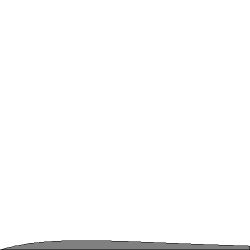## Commutativity of iterated limits

The following is a weird result I've obtained with iterated limits. There must be a flaw somewhere in someone's reasoning but I can't discover what it is. The problem is that, in general, iterated limits are not supposed to be commutative. However, the purported proof below seems to indicate that they always are. Did I make a mistake somewhere?

Let $F$ be a real valued function of two real variables defined in some region around $(a,b)$. Then the standard limit [ correct? ] of $F$ as $(x,y)$ approaches $(a,b)$ equals $L\,$ if and only if for every $\epsilon > 0$ there exists $\delta > 0$ such that $F$ satisfies: $$| F(x,y) - L | < \epsilon$$ whenever the distance between $(x,y)$ and $(a,b)$ satisfies: $$0 < \sqrt{ (x-a)^2 + (y-b)^2 } < \delta$$ We will use the following notation for such limits of functions of two variables: $$\lim_{(x,y)\rightarrow (a,b)} F(x,y) = L$$ Note. At this moment, we do not wish to consider limits where (one of) the independent variable(s) approaches infinity.

Next we consider the following iterated limit: $$\lim_{y\rightarrow b} \left[ \lim_{x\rightarrow a} F(x,y) \right] = L$$ Theorem. Commutativity of iterated limits. $$\lim_{y\rightarrow b} \left[ \lim_{x\rightarrow a} F(x,y) \right] = \lim_{x\rightarrow a} \left[ \lim_{y\rightarrow b} F(x,y) \right] = \lim_{(x,y)\rightarrow (a,b)} F(x,y)$$ Proof. We split the first iterated limit in two pieces: $$\lim_{x\rightarrow a} F(x,y) = F_a(y)$$ And: $$\lim_{y\rightarrow b} F_a(y) = L$$ Thus it becomes evident that the (first) iterated limit is actually defined as follows.
For every $\epsilon_x > 0$ there is some $\delta_x > 0$ such that: $$| F(x,y) - F_a(y) | < \epsilon_x \quad \mbox{whenever} \quad 0 < | x - a | < \delta_x$$ For every $\epsilon_y > 0$ there is some $\delta_y > 0$ such that: $$| F_a(y) - L | < \epsilon_y \quad \mbox{whenever} \quad 0 < | y - b | < \delta_y$$ Applying the triangle inequality $|a| + |b| \ge |a + b|$ gives: $$| F(x,y) - F_a(y) | + | F_a(y) - L | \ge | F(x,y) - L |$$ Consequently: $$| F(x,y) - L | < \epsilon_x + \epsilon_y$$ On the other hand we have: $$0 < | x - a | < \delta_x \qquad \mbox{and} \qquad 0 < | y - b | < \delta_y$$ Hence: $$0 < \sqrt{ (x-a)^2 + (y-b)^2 } < \sqrt{ \delta_x^2 + \delta_y^2 }$$ This is exactly the definition of the above standard limit of a function of two variables if we put: $$\epsilon = \epsilon_y + \epsilon_y \qquad \mbox{and} \qquad \delta = \sqrt{\delta_x^2 + \delta_y^2}$$ Therefore: $$\lim_{y\rightarrow b} \left[ \lim_{x\rightarrow a} F(x,y) \right] = \lim_{(x,y)\rightarrow (a,b)} F(x,y)$$ In very much the same way we can prove that: $$\lim_{x\rightarrow a} \left[ \lim_{y\rightarrow b} F(x,y) \right] = \lim_{(x,y)\rightarrow (a,b)} F(x,y)$$ QED

Rather a comment than an answer. The only thing missing in Georgy's answer is an integral: $$\int_0^\infty n^2xe^{-nx} dx = -\frac{1}{n} \int_0^\infty n^2x\, d e^{-nx} = \left[ - \frac{n^2x e^{-nx}}{n} \right]_0^\infty + \int_0^\infty n e^{-nx} dx = 0-\left[e^{-nx}\right]_0^\infty = 1$$ And a picture, where the grey area is independent of $\,n$ , i.e. the one just calculated:It is seen that Georgy's function converges to a Dirac delta at $x=0$.
This is similar to the behaviour of a simpler function at : Iterated Limits Schizophrenia